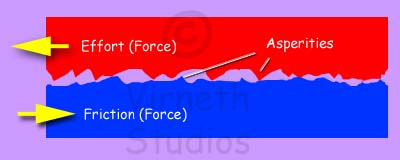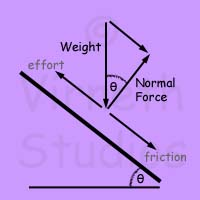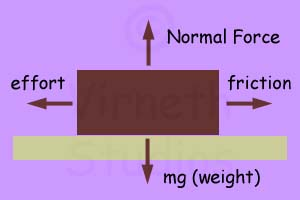# Friction

## What is Friction?

Friction is a Resistance force that opposes the relative movement (motion) of two solid objects in contact with each other. Friction is usually measured in Newtons, lb or kg depending on what units are being used to measure the Motive Effort (Push or Pull).

No surface is completely smooth. Surfaces have tiny microscopic ridges and bumps called asperities. Surfaces touch at some of these points.Friction can be caused by three things:

• Molecular adhesion (asperities sticking where they touch)

The main cause of friction is adhesion. When two surfaces touch, electromagnetic forces make molecules and atoms of both surfaces start to bond to each other at or near the contact points. This process is called adhesion. When one surface moves across another these adhesive forces have to be broken, which takes effort, known as friction. Every time a contact point is broken another one is made, so friction is always there (persistent).

• Surface roughness (asperities colliding)

As one surface slides across another the asperities can hit each other, causing abrasion by breaking the tips of the asperities. This requires effort, opposing the motive effort.

• Ploughing (a hard material digging in to a soft material)

Some materials are soft. When a hard material is moved across a soft one the hard object can dig in, causing the softer material to deform, adding to the friction.

Friction only exists when there is a motive force.

## Friction is linked to pressure

The force acting to press two surfaces together is at a right angles to them and is called the Normal Force. The Normal Force is usually measured in Newtons, lb or kg

If the object moves on an inclined plane (a slope) then the Normal force is a component of the weight.

Nf = Cosθ.WfWhen the movement of one object over another is horizontal the Normal Force is the same as the weight of the top object.

As the size of the Normal Force gets bigger so does the friction between the surfaces. This is because the higher force presses the surfaces closer together and increases the number of asperities that are in contact.

So Friction is proportional to the Normal Force. (Try it below - add the extra weight)

If you increase the size of the contact area the friction does not go up. This is because there are still only about the same number of asperities in contact with each other.

## Co-Efficient of Friction (μ).

Friction is mainly due to interactions between lots of charged particles (e.g. atoms and molecules). This makes it impossible to calculate directly the friction produced by two materials.

Instead two materials are tested with different values of Normal Force (Nf). The results are used to find a value that defines the ratio between friction and the Normal force (Nf). This value is called  the co-efficient of friction and is identified by the symbol μ (Greek letter mu).

The Co-efficient of Friction can then be used in a general expression to find the friction

Ff = μ N                Friction = Co-efficient of friction of two materials (μ) multiplied by the normal force (N).

Each type of material behaves differently with other materials. For example the friction between rubber and steel will be different to the friction between steel against brass. From testing we end up with a table of co-efficients (see below). These values are really only for general reference. In practice material properties can vary greatly with temperature, humidity etc. and, before specific materials are used in real situations, it is usual for samples to be tested under actual conditions.

## Static and Kinetic Friction

There are two types of friction:

1. Static Friction (μs): The friction between static objects. It is the force neccessary to start a body moving.

2. Kinetic Friction (μk): The friction between moving objects. It is the minimum force required to keep a body moving. It is less than the static force. Kinetic Friction produces heat from the process of breaking the particle bonding between the surfaces.

Friction can be found using the expression: Ff = μ Nf

where

Ff = frictional force (N, lb or kg)

μ = static (μs) or kinetic (μk) co-efficient of friction

Nf = normal force (N, lb or kg)

For an object that is moved horizontally, the normal force (Nf) is the weight: Nf = m g

where

m = mass of the object (kg, lbs)

g = acceleration of gravity (9.81 m/s2, 32.19 ft/s2)

## Application of Friction

Friction can be used to advantage. For example in clutches that regulate the amount of power transmitted from a car engine to the wheels, or when walking. More>

## Lubrication and Bearings

Friction causes heat and wear. This can cost a lot of money when having to replace items that have worn out. More>

(These figures are a rough guide of some of the more common co-efficients of friction. In practice the actual co-efficient of friction of two specific materials should be found by trial)

## Kinetic (μk)

Aluminium Aluminium 1.2 1.4
Aluminium Steel 0.61 0.47
Brass Cast Iron   0.3
Bronze Cast Iron   0.22
Cast Iron Cast Iron 1.1 0.15
Cast Iron Copper 1.05 0.29
Copper Copper 1.0
Copper Steel 0.53 0.36
Iron Iron 1.0
Leather Wood 0.35
Leather Metal 0.6
Nylon Nylon 0.2
Rubber Asphalt   0.65
Steel Brass 0.51 0.44
Steel Cast Iron   0.23